Differential Calculus NAT Level - 2

# Differential Calculus NAT Level - 2

Test Description

## 10 Questions MCQ Test Topic wise Tests for IIT JAM Physics | Differential Calculus NAT Level - 2

Differential Calculus NAT Level - 2 for IIT JAM 2023 is part of Topic wise Tests for IIT JAM Physics preparation. The Differential Calculus NAT Level - 2 questions and answers have been prepared according to the IIT JAM exam syllabus.The Differential Calculus NAT Level - 2 MCQs are made for IIT JAM 2023 Exam. Find important definitions, questions, notes, meanings, examples, exercises, MCQs and online tests for Differential Calculus NAT Level - 2 below.
Solutions of Differential Calculus NAT Level - 2 questions in English are available as part of our Topic wise Tests for IIT JAM Physics for IIT JAM & Differential Calculus NAT Level - 2 solutions in Hindi for Topic wise Tests for IIT JAM Physics course. Download more important topics, notes, lectures and mock test series for IIT JAM Exam by signing up for free. Attempt Differential Calculus NAT Level - 2 | 10 questions in 45 minutes | Mock test for IIT JAM preparation | Free important questions MCQ to study Topic wise Tests for IIT JAM Physics for IIT JAM Exam | Download free PDF with solutions
 1 Crore+ students have signed up on EduRev. Have you?
*Answer can only contain numeric values
Differential Calculus NAT Level - 2 - Question 1

### Maximum area of a rectangle which can be inscribed in a circle of given radius R is given by αR2. Find the value of α.

Detailed Solution for Differential Calculus NAT Level - 2 - Question 1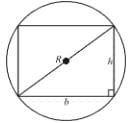Let rectangle has width b and height h.
Area = h·b
Also,  b2 + h2 = (2R)2 = 4R2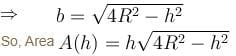Area is maximum when A2 is maxima
A2 = h2(4R2 – h2)]
f(h) = h2(4R2 – h2)
For maxima,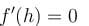⇒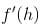= h2(–2h) + (4R2 – h2)2h = 0

h2 + 4R2 – h2 = 0
h2 = 2R2
h = √2

From physical nature of problem, it is clear that this should be maximum area since minimum area will tend towards zero.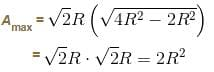Hence, value of α = 2

*Answer can only contain numeric values
Differential Calculus NAT Level - 2 - Question 2

### The radius of a right circular cylinder increases at a constant rate. Its altitude is a linear function of the radius and increases three times as fast as radius. When the radius is 1 cm the altitude is 6 cm. When the radius is 6 cm, the volume is increasing at the rate of 1 cm/s. When the radius is 36 cm, the volume is increasing at a rate of n cm3/s. The value of 'n' is equal to :

Detailed Solution for Differential Calculus NAT Level - 2 - Question 2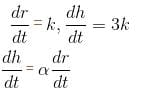h = αr + c
α = 3
h = 3r + c
h = 6, r = 1
c = 3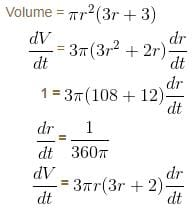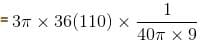= 33 cm3/second

*Answer can only contain numeric values
Differential Calculus NAT Level - 2 - Question 3

### The maximum value of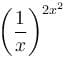is given as (λ/e). The value of  λ is

Detailed Solution for Differential Calculus NAT Level - 2 - Question 3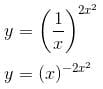⇒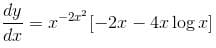For maxima or minima of y.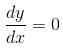⇒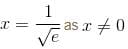and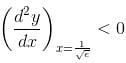Hence, y attains maximum value at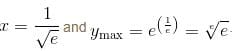The value of λ = 1.

*Answer can only contain numeric values
Differential Calculus NAT Level - 2 - Question 4

Consider the function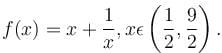If α is the length of interval of decrease and β be the length of interval of increase, then β/α is

Detailed Solution for Differential Calculus NAT Level - 2 - Question 4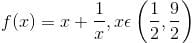f(x) is decreasing in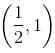and increasing in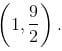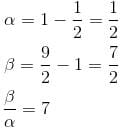*Answer can only contain numeric values
Differential Calculus NAT Level - 2 - Question 5

The ratio of absolute maxima and minima of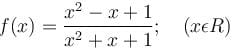is

Detailed Solution for Differential Calculus NAT Level - 2 - Question 5

Absolute Maxima = 3
and The Absolute Minima = 1/3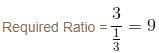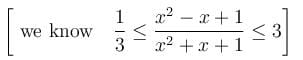*Answer can only contain numeric values
Differential Calculus NAT Level - 2 - Question 6

If the interval of monotonicity of the function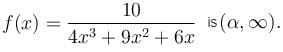Find the value of  α?

Detailed Solution for Differential Calculus NAT Level - 2 - Question 6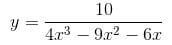⇒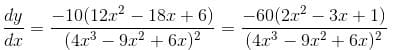⇒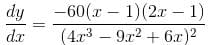⇒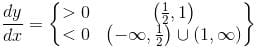Hence,    value of α = 1

*Answer can only contain numeric values
Differential Calculus NAT Level - 2 - Question 7

The least area of a circle circumscribing any right triangle of area S is given as απS. Find the value of α.

Detailed Solution for Differential Calculus NAT Level - 2 - Question 7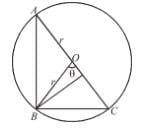Area of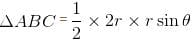S = r2sin θ
⇒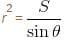Area of circle =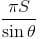Least area = πS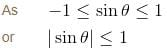So,               Value of α = 1

*Answer can only contain numeric values
Differential Calculus NAT Level - 2 - Question 8

Let  f(x) = 2x3 + ax2 + bx - 3cos2 x is an increasing function for all  x∈R  such that  ma2 + nb + 18 < 0 then the value of m + n + 7 is

Detailed Solution for Differential Calculus NAT Level - 2 - Question 8

Given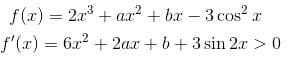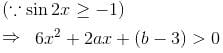D < 0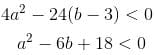m = 1, n = – 6
m + n + 7 = 1 – 6 + 7
= 8 – 6 = 2

*Answer can only contain numeric values
Differential Calculus NAT Level - 2 - Question 9

If the maximum value of the function f(x) = (sin-1 x)3 + (cos-1 x)3, -1 << 1 is α and minimum value is β and α - β is of the form n · π3. Find the value of n.

Detailed Solution for Differential Calculus NAT Level - 2 - Question 9

Let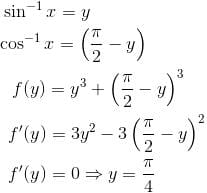π/4  is point of minima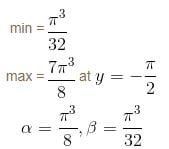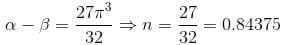*Answer can only contain numeric values
Differential Calculus NAT Level - 2 - Question 10

If a, b, c, d are real numbers such that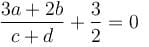then the equation ax3 + bx2 + cx + d = 0  has at least one root in (0, α). Find the value of α.

Detailed Solution for Differential Calculus NAT Level - 2 - Question 10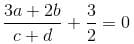⇒  6a + 4b + 3c + 3d = 0
Let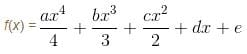f(0) = e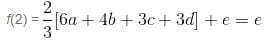Since f(x) is continuous and differentiable in (0,2) and f(0) = f(2) = e

Hence, according to Rolle's Theorem, the equation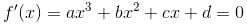has at least one root in (0,2). Thus, value of α = 2.

## Topic wise Tests for IIT JAM Physics

217 tests
Information about Differential Calculus NAT Level - 2 Page
In this test you can find the Exam questions for Differential Calculus NAT Level - 2 solved & explained in the simplest way possible. Besides giving Questions and answers for Differential Calculus NAT Level - 2, EduRev gives you an ample number of Online tests for practice

## Topic wise Tests for IIT JAM Physics

217 tests(Scan QR code)